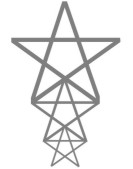All about flooble | fun stuff | Get a free chatterbox | Free JavaScript | Avatarsperplexus dot infoStar Stack (Posted on 2004-04-12)Three pentagram-shaped stars (the stars formed from the diagonals of a regular pentagon) are stacked up so that the bottom two ends of the tips touch the middle ends of the tips of the star below. (See diagram.)

The distance from the top of the stack to the floor (where the bottom star's "feet" rest) is 4 feet.

What is the distance between the bottom two ends of the tips of the stack that touch the floor?

 No Solution Yet Submitted by Gamer Rating: 3.5000 (6 votes)Comments: ( Back to comment list | You must be logged in to post comments.)different answer| Comment 5 of 19 |First thing to note is that for all stars the pentagon is regular
and the triangles (i.e., the "points" of the star) are 72-36-72 isoscoles.

Let a side (i.e., one of the five straight lines connecting two of the star's outer points) of the big pentagram be denoted by s(big) = s.

And using simple trig then the height and the base of the big star are,

h(big) = s*sin72
b(big) = 2s*cos72.

Find the side, height and base of the medium pentagram.

s(med) = b(big) = 2s*cos72
h(med) = h(big) * s(med)/s = 2s*cos72*sin72
b(med) = b(big) * s(med)/s = 4s*cos72*cos72

Find the side, height and base of the little pentagram.

s(lit) = b(med) = 4s*cos72*cos72
h(lit) = h(med) * s(lit)/s(med) = 4s*cos72*cos72*sin72
b(lit) = b(med) * s(lit)/s(med) = 8s*cos72*cos72*cos72

We're told the stacked height is 4. I.e., h(lit) + h(med) + h(big) = 4.

This gives a solution for s.

4s*cos72*cos72*sin72 + 2s*cos72*sin72 + s*sin72 = 4

s = 4/(4cos72*cos72*sin72 + 2cos72*sin72 + sin72)

We want to find b(lit).

b(lit) = 8s*cos72*cos72*cos72

substituting for s

b(lit) = 32cos72*cos72*cos72/(4cos72*cos72*sin72 + 2cos72*sin72 + sin72)

b(lit) = 0.4964221213 feet.

 Posted by vectorboy on 2004-05-22 17:39:14Please log in:

 Search: Search body:
Forums (2)